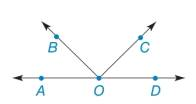Chapter 1.3, Problem 19E### Elementary Geometry for College St...

6th Edition
Daniel C. Alexander + 1 other
ISBN: 9781285195698

#### Solutions

Chapter
Section### Elementary Geometry for College St...

6th Edition
Daniel C. Alexander + 1 other
ISBN: 9781285195698
Textbook Problem
1 views

# In Exercises 9 to 28, use the drawings as needed to answer the following questions.In the figure. namea) two opposite rays.b) two rays that are not opposite.To determine

a)

To find:

Two opposite rays.

Explanation

Given:

The given figure is,

Definition:

If the two rays have a common endpoint and the union of those rays is a straight line, then the rays are said to be opposite rays.

Approach:

Consider the given figure,

Figure (1)

In the given figure, rays OAandOD have

To determine

b)

To find:

Two rays that is not opposite.

### Still sussing out bartleby?

Check out a sample textbook solution.

See a sample solution

#### The Solution to Your Study Problems

Bartleby provides explanations to thousands of textbook problems written by our experts, many with advanced degrees!

Get Started

#### Find more solutions based on key concepts